# Evaluating Off-the-Shelf Optical Components

Off-the-shelf optical components offer a number of advantages for optical system design, including shorter delivery times, lower costs, and quicker replacement. These readily available optical products, however, are seldom used in custom optical designs. One reason is that no clear or uniform methodology exists to determine how effectively such optics will perform in a real-world optical instrument.

A common way to use off-the-shelf optical components is in metrology test stands, where there are no volume restrictions. Off-the-shelf lenses work reasonably well in applications requiring low numerical apertures (NAs) and small field angles and field sizes. When applying off-the-shelf components in manufactured optical systems, however, lens effectiveness plays a more critical role in optimizing the overall optical system performance.

One comparatively straightforward approach to measuring how effectively standard lens components — specifically, single lenses, cemented doublets, and triplets — will perform is to combine a lens’s glass refraction index and Abbe number. Factoring both of these features together provides users with a single parameter by which to evaluate the level of monochromatic and chromatic lens aberration and thereby predict image quality.

Demonstrations of this method follow. All are based on evaluations of off-the-shelf optical components that are made from various glass materials but that possess identical first-order optical parameters — for example, focal length, number of lenses, or NA. These evaluations apply a new optical parameter, the value of which helps to define the image quality or residual aberrations that an optical component exhibits. Components that demonstrate higher parameter values contribute lower aberrations, making them more suitable for use in custom optical system designs.

Three formulas

The refractive glass index and Abbe number are the two most critical glass constants when evaluating the effectiveness of off-the-shelf lenses. The composition of these constants defines aberration correction.

Lenses with a high refractive index value (for example, >1.75) are effective for correcting monochromatic aberrations, while lenses with a high Abbe number (for example, >80) can help to correct chromatic aberrations.

### Comparative Data of Off-the-Shelf Optical Components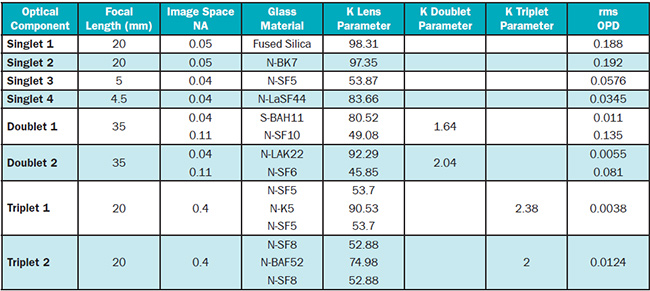NA: numerical aperture; rms OPD: root mean square optical path difference.

The lens parameter proposed for defining lens effectiveness comprises both of these glass constants, and it is defined as K in the following formula: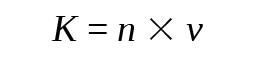where n is the glass refractive index and v is the Abbe number. For a single lens, K is thoroughly defined by the formula.

Cemented glass doublets that consist of two lenses are described by two K parameters: one for the crown glass, which has positive optical power, and the other for the flint glass, which has negative optical power. The cemented doublet parameter (Kdb) is defined by the following formula: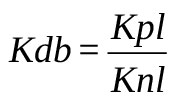where Kpl is the K parameter for the lens with positive power, and Knl is the K parameter for the lens with negative power.

The parameter for cemented doublets is defined this way because, if both doublet lenses were made from the same glass, Kdb = 1, there would be no difference between the doublet and singlet lenses. Even so, in this case, the doublet’s effectiveness parameter is equivalent to the K parameter of a single lens.

The cemented triplet lens component presents a unique case. The lens can be considered to be two cemented doublets, where two lenses are made from the same glass. So, this would make a total of three glasses. But we can operate with two doublet lenses. The K parameter for a cemented triplet lens component is defined by the following formula: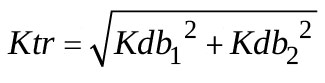where Kdb1 and Kdb2 are K doublet parameters located inside a cemented triplet lens, and the cemented triplet’s effectiveness is expressed by the parameter Ktr.

Using these three formulas allows the calculation of the effectiveness parameter for any optical lens component. It also allows comparison between optical components that share the same optical characteristics but are fabricated from different optical glasses.

Practical implementation

Practical implementation of this method can be illustrated by evaluating the same three types of off-the-shelf lens components: single lenses, cemented doublets, and cemented triplets. All lens data was taken from Thorlabs and Edmund Optics product catalogs. Each group of optical components shares the same first-order design data, but each was made from different glasses. The table tabulates the data for all optical components, their working conditions, and their calculated lens K parameters.

The K parameters shown for single lenses, doublets, and triplets are defined by formulas 1, 2, and 3, respectively. In the last column, the root mean square (rms) optical path difference (OPD) was derived from the results of each optical component’s aberration analyses. These results show a direct relation between the K parameter value for each optical component and the quality of the wavefront (wave aberration) in the image plane. The Singlet 1 and Singlet 2 lens products produced similar K parameter values, but their rms OPDs show the small difference in wavefront aberration. This means that the method described above has very high sensitivity.

The results for cemented doublet lens components were calculated from two image space NA values. For a more complete analysis, the polychromatic aberration graph data would be used for the N-SF5, N-LaSF44, and S-BAH11 glass materials.

Figure 1 represents wave aberration plots for singlet lenses with different K parameter values. The lens with the larger K value (K = 83.66) offers lower wave aberration and better image quality. All other factors being equal, this gives the lens an advantage when used as a single component or as part of complex optical system, where it would help to minimize the system’s total aberration1.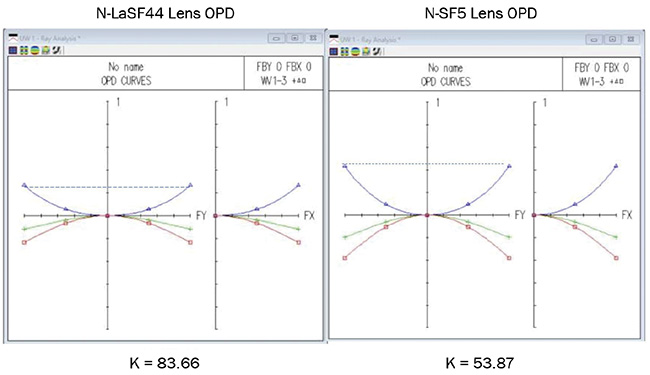Figure 1. Wave aberration plots for two singlet lenses. Courtesy of Lev Ryzhikov.

Figure 2 demonstrates aberration plots for two doublets used in the evaluation. Both doublets have the same optical characteristics but are made from different glasses. Consequently, their K doublet parameters are different.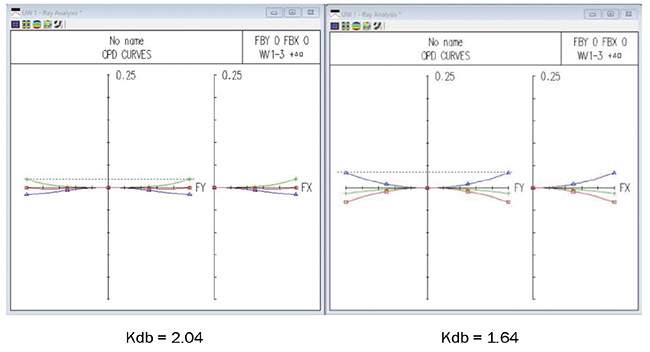Figure 2. Wave aberration plots for two doublet lens components. Courtesy of Lev Ryzhikov.

Figure 3 plots the wave aberrations for the off-the-shelf cemented triplets. As illustrated by the evaluated singlet and doublet lenses, triplet lens components with larger K parameters exhibited smaller wave aberrations, signifying more favorable performance when used as components in custom optical systems.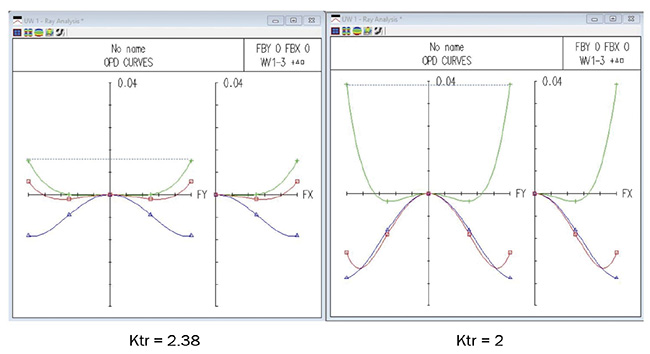Figure 3. Wave aberration plots for two triplet lens components. Courtesy of Lev Ryzhikov.

Thus, it is possible to assert that the K parameter for any single optical lens component uniquely identifies its capability to correct aberrations. Advanced lens design software offers the further possi­bility to optimize the shape and thickness of lens components. But choosing the proper glass is also important because it allows for dramatic improvements to overall system performance. The proposed method for evaluating the perfor­mance of off-the-shelf lenses can be applied to derive the K parameter for virtually any type of optical component. But applying it to evaluate off-the-shelf lenses may allow them to be used as a substitution for some custom lenses, enabling the shorter delivery times, lower costs, and faster replacement times possible when ordering from a lens product catalog. The method described can also be used to evaluate high-NA objective lens systems to help find proper lens components.

Meet the author

Lev Ryzhikov is a retired optical engineer; email: [email protected].

Reference

1. G.G. Slyusarev (1984). Aberration and Optical Design Theory, 2nd ed. Boca Raton, Fla.: CRC Press.

Photonics Spectra
Jun 2021
columnsPhotonic FundamentalsopticslensesimagingTest & Measurement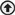view all
xSubscribe to Photonics Spectra magazine - FREE!